Courses

# Test: Rectangular Solids- 3

## 10 Questions MCQ Test Quantitative Aptitude for GMAT | Test: Rectangular Solids- 3

Description
This mock test of Test: Rectangular Solids- 3 for GMAT helps you for every GMAT entrance exam. This contains 10 Multiple Choice Questions for GMAT Test: Rectangular Solids- 3 (mcq) to study with solutions a complete question bank. The solved questions answers in this Test: Rectangular Solids- 3 quiz give you a good mix of easy questions and tough questions. GMAT students definitely take this Test: Rectangular Solids- 3 exercise for a better result in the exam. You can find other Test: Rectangular Solids- 3 extra questions, long questions & short questions for GMAT on EduRev as well by searching above.
QUESTION: 1

### A 5 cubic centimeter cube is painted on all its side. If it is sliced into 1 cubic centimer cubes, how many 1 cubic centimeter cubes will have exactly one of their sides painted?

Solution:

When a 5 cc cube is sliced into 1 cc cubes, we will get 5*5*5 = 125 1 cc cubes.

In each side of the larger cube, the smaller cubes on the edges will have more than one of their sides painted.
Therefore, the cubes which are not on the edge of the larger cube and that lie on the facing sides of the larger cube will have exactly one side painted.

In each face of the larger cube, there will be 5*5 = 25 cubes. Of these, there will be 16 cubes on the edge and 3*3 = 9 cubes which are not on the edge.
Therefore, there will be 9 1-cc cubes per face that will have exactly one of their sides painted.
In total, there will be 9*6 = 54 such cubes.

QUESTION: 2

### The area of a square field is 24200 sq m. How long will a lady take to cross the field diagonally at the rate of 6.6 km/hr?

Solution:

Let ‘a’ meters be the length of a side of the square field.
Therefore, its area = a2 square meters. --- (1)
We know that the length of the diagonal ‘d’ of a square whose side is ‘a’ meters =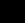a –-- (2)
From (1) and (2), we can deduce that the square of the diagonal = d2 = 2a2

Or d =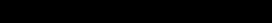meters.

The time taken to cross a length of 220 meters while traveling at 6.6 kmph is given by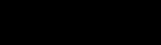(converting 1 km = 1000 meters and 1 hour = 60 minutes).= 2 minutes

QUESTION: 3

### The circumference of the front wheel of a cart is 30 ft long and that of the back wheel is 36 ft long. What is the distance travelled by the cart, when the front wheel has done five more revolutions than the rear wheel?

Solution:

The circumference of the front wheel is 30 ft and that of the rear wheel is 36 feet.

Let the rear wheel make n revolutions. At this time, the front wheel should have made n+5 revolutions.

As both the wheels would have covered the same distance, n*36 = (n+5)*30

36n = 30n + 150
6n = 150
n = 25.

Distance covered = 25*36 = 900 ft.

QUESTION: 4

If the sides of a triangle measure 72, 75 and 21, what is the measure of its in radius?

Solution:

The sides of the triangle happen to be a Pythagorean triplet. Hence, the triangle is a right angled triangle.

For a right angled triangle,

The measure of in radius = (product of perpendicular sides) / (perimeter of triangle)

= (72 * 21) / (72 + 21 +75) = 1512 / 168 = 9

QUESTION: 5

A 5 cm cube is cut into as many 1 cm cubes as possible. What is the ratio of the surface area of the larger cube to that of the sum of the surface areas of the smaller cubes?

Solution:

The volume of the larger cube = 53 = 125 cm3.
The volume of each of the smaller cubes = 13 = 1 cm3. Therefore, one would get 125 smaller cubes.
The surface area of the larger cube = 6a2 = 6(52) = 6 * 25 = 150
The surface area of each of the smaller cubes = 6 (12) = 6.
Therefore, surface area of all of the 125, 1 cm3 cubes = 125 * 6 = 750.
Therefore, the required ratio = 150 : 750 = 1 : 5

QUESTION: 6

If each interior angle of a regular polygon is 150 degrees, then it is a/an ______________

Solution:

The sum of the exterior angle and interior angle of a regular polygon is 3600.
As the measure of each interior angle is 1500, the measure of each exterior angle will be 300.
The number of sides of a regular polygon is given by the following relationship n = 360/exterior angle
As the value of each exterior angle is 300, the number of sides = 12.

QUESTION: 7

Four horses are tethered at 4 corners of a square field of side 70 metres so that they can just about reach one another. The area left ungrazed by the horses is:

Solution:

The length of the rope in which the horses tied should be equal to half of the side of the square plot so that they just cannot reach one another.
Therefore, the length of the rope is 35m (70/2).
The area covered by each horse should be equal to the area of sector with radius of 70/2 = 35m(length of the rope).
Total area covered by the four horses = 4* area of sector of radius 35 metres = Area of circle of radius 35m.
Area left ungrazed by the horses = Area of square field - Area covered by four horses.
= 702 - (22/7)*35*35 = 4900 - 3850 = 1050 sq.m.

QUESTION: 8

A square sheet of paper is converted into a cylinder by rolling it along its length. What is the ratio of the base radius to the side of the square?

Solution:

Surface area of the cylinder = Surface area of the square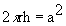Here height 'h' of the cylinder = side of the square since it is rolled along its length.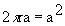Base radius r =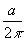Ratio of base radius to side of square =: a =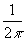QUESTION: 9

The surface area of the three coterminous faces of a cuboid are 6, 15, 10 sq.cm respectively. Find the volume of the cuboid.

Solution:

If l, b, h be the dimensions of the cuboid, then volume of the cuboid = l X b X h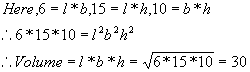QUESTION: 10

If the diagonal and the area of a rectangle are 25 m and 168 m2, what is the length of the rectangle?

Solution:

The diagonal d = 25m. and area A = 168 m2.
Let 'l' be the length and 'b' be the width of the rectangle.
Therefore, l2 + b2 = d2. and lb = A
We can therefore write (l + b)2 = d2 + 2A and (l - b)2 = d2 - 2A.
Substituting and solving we get, l + b = 31 and l - b = 17. Hence l = 24 and b = 7